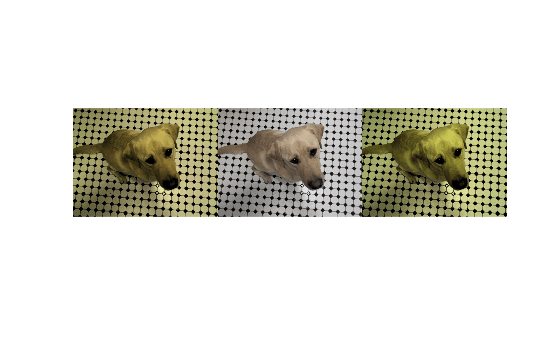# jitterColorHSV

Randomly alter color of pixels

Since R2019b

## Syntax

``J = jitterColorHSV(I,Name=Value)``

## Description

example

````J = jitterColorHSV(I,Name=Value)` adjusts the color of RGB image `I` with a randomly selected value of hue, saturation, brightness, and contrast from the HSV color space. Specify the range of each type of adjustment using name-value arguments.```

## Examples

collapse all

```I = imread("kobi.png"); imshow(I)```Randomly adjust the hue, saturation, brightness, and contrast of the image. To demonstrate the randomness of the adjustment, repeat the operation on the original image three times.

```J1 = jitterColorHSV(I,Contrast=0.4,Hue=0.1,Saturation=0.2,Brightness=0.3); J2 = jitterColorHSV(I,Contrast=0.4,Hue=0.1,Saturation=0.2,Brightness=0.3); J3 = jitterColorHSV(I,Contrast=0.4,Hue=0.1,Saturation=0.2,Brightness=0.3);```

Display the adjusted images in a montage.

`montage({J1,J2,J3},Size=[1 3])`## Input Arguments

collapse all

RGB image with original pixel values, specified as an m-by-n-by-3 numeric array.

Data Types: `single` | `double` | `uint8` | `uint16`

### Name-Value Arguments

Specify optional pairs of arguments as `Name1=Value1,...,NameN=ValueN`, where `Name` is the argument name and `Value` is the corresponding value. Name-value arguments must appear after other arguments, but the order of the pairs does not matter.

Example: `J = jitterColorHSV(I,Hue=0.1)` adds a random amount of hue from the uniform distribution [-0.1, 0.1].

Before R2021a, use commas to separate each name and value, and enclose `Name` in quotes.

Example: `J = jitterColorHSV(I,"Hue",0.1)` adds a random amount of hue from the uniform distribution [-0.1, 0.1].

Hue offset, specified as one of the following values. `jitterColorHSV` converts input RGB image `I` to the HSV color space before adding a random value to the hue channel of the image. `jitterColorHSV` circularly wraps the modified hue to the range [0, 1] before converting the jittered HSV image back to the RGB color space.

ValueMeaning
Numeric scalar in the range [0, 1]Add random amount of hue from the uniform distribution ```[-Hue Hue]```
2-element numeric vector with elements in the range [-1, 1]Add a random amount of hue from a continuous uniform distribution within the specified interval. The second element must be larger than or equal to the first element.

Data Types: `single` | `double`

Saturation offset, specified as one of the following values. `jitterColorHSV` converts input RGB image `I` to the HSV color space before adding a random value to the saturation channel of the image. `jitterColorHSV` clips the modified saturation to the range [0, 1] before converting the jittered HSV image back to the RGB color space.

ValueMeaning
Numeric scalar in the range [0, 1]Add random amount of saturation from the uniform distribution `[-Saturation Saturation]`
2-element numeric vector with elements in the range [-1, 1]Add a random amount of saturation from a continuous uniform distribution within the specified interval. The second element must be larger than or equal to the first element.

Data Types: `single` | `double`

Brightness offset, specified as one of the following values. `jitterColorHSV` converts input RGB image `I` to the HSV color space before adding a random value to the brightness (value) channel of the image. `jitterColorHSV` clips the modified brightness to the range [0, 1] before converting the jittered HSV image back to the RGB color space.

ValueMeaning
Numeric scalar in the range [0, 1]Add random amount of brightness from the uniform distribution `[-Brightness Brightness]`
2-element numeric vector with elements in the range [-1, 1]Add a random amount of brightness from a continuous uniform distribution within the specified interval. The second element must be larger than or equal to the first element.

Data Types: `single` | `double`

Contrast scale factor, specified as one of the following values. `jitterColorHSV` converts input RGB image `I` to the HSV color space before scaling the brightness (value) channel of the image by a random factor. `jitterColorHSV` clips the modified brightness to the range [0, 1] before converting the jittered HSV image back to the RGB color space.

ValueMeaning
Positive numberScale the brightness by a random factor from the uniform distribution `[1-Contrast 1+Contrast]`
2-element numeric vector of positive numbersScale the brightness by a random factor from the uniform distribution within the specified interval. The second element must be larger than or equal to the first element.

Data Types: `single` | `double`

## Output Arguments

collapse all

Jittered RGB image, returned as a numeric array of the same size and data type as the input image, `I`.

Data Types: `single` | `double` | `uint8` | `uint16`

collapse all

### HSV Color Space

The HSV color space defines the hue, saturation, and value (brightness) for each pixel, respectively, as described in the table.

AttributeDescription
HueValue from `0` to `1` that corresponds to the color’s position on a color wheel. As hue increases from `0` to `1`, the color transitions from red to orange, yellow, green, cyan, blue, magenta, and finally back to red.
SaturationAmount of hue or departure from neutral. `0` indicates a grayscale image and `1` indicates maximum saturation.
Value

Maximum value among the red, green, and blue components of a specific color.

## Version History

Introduced in R2019b